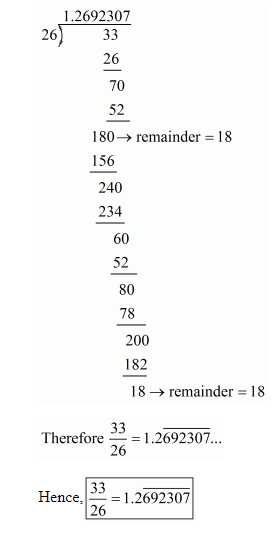# Express the following rational numbers as decimals:`
Question:

Express the following rational numbers as decimals:

(i) $\frac{2}{3}$

(ii) $-\frac{4}{9}$

(iii) $\frac{-2}{15}$

(iv) $-\frac{22}{13}$

(v) $\frac{437}{999}$

(vi) $\frac{33}{26}$

Solution:

(i) Given rational number is $\frac{2}{3}$

Now we have to express this rational number into decimal form. So we will use long division method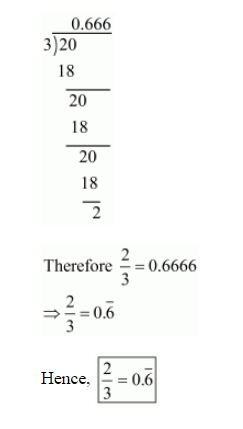(ii) Given rational number is $-\frac{4}{9}$

Now we have to express this rational number into decimal form. So we will use long division method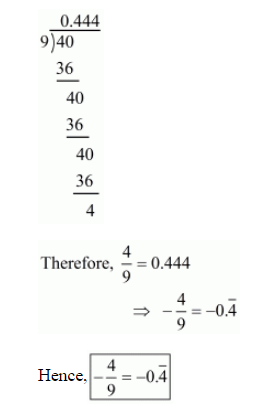(iii) Given rational number is $-\frac{2}{15}$

Now we have to express this rational number into decimal form. So we will use long division method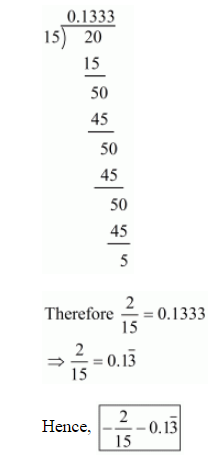(iv) Given rational number is $-\frac{22}{13}$

Now we have to express this rational number into decimal form. So we will use long division method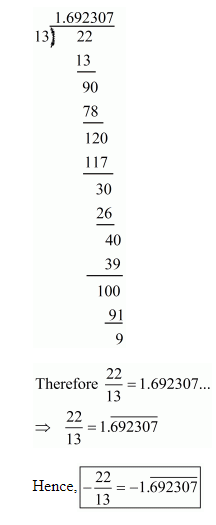(v) Given rational number is $\frac{437}{999}$

Now we have to express this rational number into decimal form. So we will use long division method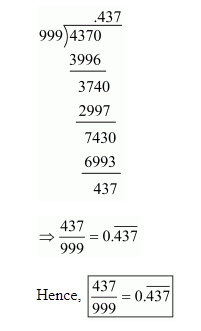(vi) Given rational number is $\frac{33}{26}$

Now we have to express this rational number into decimal form. So we will use long division method